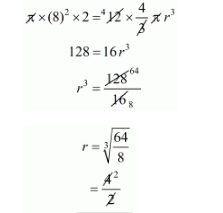# 12 spheres of the same size are made from melting`
Question:

12 spheres of the same size are made from melting a solid cylinder of 16 cm diameter and 2 cm height. The diameter of each sphere is

(a) $\sqrt{3} \mathrm{~cm}$

(b) 2 cm

(c) 3 cm

(d) 4 cm

Solution:

The volume of solid cylinder = 12 × volume of one sphere$r=2 \mathrm{~cm}$

The required diameter d = 2 × 2 = 4 cm

Hence, the correct answer is choice (d).